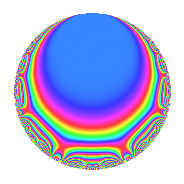# Properties

 Label 4020.2.q.iLevel 4020 Weight 2 Character orbit 4020.q Analytic conductor 32.100 Analytic rank 0 Dimension 4 CM No Inner twists 2

# Related objects

## Newspace parameters

 Level: $$N$$ = $$4020 = 2^{2} \cdot 3 \cdot 5 \cdot 67$$ Weight: $$k$$ = $$2$$ Character orbit: $$[\chi]$$ = 4020.q (of order $$3$$ and degree $$2$$)

## Newform invariants

 Self dual: No Analytic conductor: $$32.0998616126$$ Analytic rank: $$0$$ Dimension: $$4$$ Relative dimension: $$2$$ over $$\Q(\zeta_{3})$$ Coefficient field: $$\Q(\sqrt{-3}, \sqrt{-11})$$ Coefficient ring: $$\Z[a_1, \ldots, a_{13}]$$ Coefficient ring index: $$3$$ Sato-Tate group: $\mathrm{SU}(2)[C_{3}]$

## $q$-expansion

Coefficients of the $$q$$-expansion are expressed in terms of a basis $$1,\beta_1,\beta_2,\beta_3$$ for the coefficient ring described below. We also show the integral $$q$$-expansion of the trace form.

 $$f(q)$$ $$=$$ $$q + q^{3} - q^{5} + ( -\beta_{1} + \beta_{3} ) q^{7} + q^{9} +O(q^{10})$$ $$q + q^{3} - q^{5} + ( -\beta_{1} + \beta_{3} ) q^{7} + q^{9} + ( \beta_{1} + \beta_{3} ) q^{11} + ( 1 - \beta_{1} ) q^{13} - q^{15} + ( -4 + 5 \beta_{1} + \beta_{2} - \beta_{3} ) q^{17} + ( -4 + 3 \beta_{1} - \beta_{2} + \beta_{3} ) q^{19} + ( -\beta_{1} + \beta_{3} ) q^{21} + ( -4 + 5 \beta_{1} + \beta_{2} - \beta_{3} ) q^{23} + q^{25} + q^{27} + ( -\beta_{1} - \beta_{3} ) q^{29} + ( -\beta_{1} - 2 \beta_{3} ) q^{31} + ( \beta_{1} + \beta_{3} ) q^{33} + ( \beta_{1} - \beta_{3} ) q^{35} + ( -2 - \beta_{1} - 3 \beta_{2} + 3 \beta_{3} ) q^{37} + ( 1 - \beta_{1} ) q^{39} + ( 7 \beta_{1} + \beta_{3} ) q^{41} + ( 1 + \beta_{2} ) q^{43} - q^{45} + ( 5 \beta_{1} - \beta_{3} ) q^{47} + ( -1 + 2 \beta_{1} + \beta_{2} - \beta_{3} ) q^{49} + ( -4 + 5 \beta_{1} + \beta_{2} - \beta_{3} ) q^{51} + ( -4 - 2 \beta_{2} ) q^{53} + ( -\beta_{1} - \beta_{3} ) q^{55} + ( -4 + 3 \beta_{1} - \beta_{2} + \beta_{3} ) q^{57} + ( 2 - 2 \beta_{2} ) q^{59} + ( -11 + 11 \beta_{1} ) q^{61} + ( -\beta_{1} + \beta_{3} ) q^{63} + ( -1 + \beta_{1} ) q^{65} + ( 6 - 6 \beta_{1} - \beta_{2} - \beta_{3} ) q^{67} + ( -4 + 5 \beta_{1} + \beta_{2} - \beta_{3} ) q^{69} + ( -3 \beta_{1} - 3 \beta_{3} ) q^{71} + ( 13 - 13 \beta_{1} ) q^{73} + q^{75} + ( -8 + 7 \beta_{1} - \beta_{2} + \beta_{3} ) q^{77} + ( 11 \beta_{1} - 2 \beta_{3} ) q^{79} + q^{81} + ( -3 \beta_{1} - 3 \beta_{2} + 3 \beta_{3} ) q^{83} + ( 4 - 5 \beta_{1} - \beta_{2} + \beta_{3} ) q^{85} + ( -\beta_{1} - \beta_{3} ) q^{87} + ( -2 - 4 \beta_{2} ) q^{89} + \beta_{2} q^{91} + ( -\beta_{1} - 2 \beta_{3} ) q^{93} + ( 4 - 3 \beta_{1} + \beta_{2} - \beta_{3} ) q^{95} + ( 1 - \beta_{1} ) q^{97} + ( \beta_{1} + \beta_{3} ) q^{99} +O(q^{100})$$ $$\operatorname{Tr}(f)(q)$$ $$=$$ $$4q + 4q^{3} - 4q^{5} - q^{7} + 4q^{9} + O(q^{10})$$ $$4q + 4q^{3} - 4q^{5} - q^{7} + 4q^{9} + 3q^{11} + 2q^{13} - 4q^{15} - 9q^{17} - 7q^{19} - q^{21} - 9q^{23} + 4q^{25} + 4q^{27} - 3q^{29} - 4q^{31} + 3q^{33} + q^{35} - q^{37} + 2q^{39} + 15q^{41} + 2q^{43} - 4q^{45} + 9q^{47} - 3q^{49} - 9q^{51} - 12q^{53} - 3q^{55} - 7q^{57} + 12q^{59} - 22q^{61} - q^{63} - 2q^{65} + 13q^{67} - 9q^{69} - 9q^{71} + 26q^{73} + 4q^{75} - 15q^{77} + 20q^{79} + 4q^{81} + 3q^{83} + 9q^{85} - 3q^{87} - 2q^{91} - 4q^{93} + 7q^{95} + 2q^{97} + 3q^{99} + O(q^{100})$$

Basis of coefficient ring in terms of a root $$\nu$$ of $$x^{4} - x^{3} - 2 x^{2} - 3 x + 9$$:

 $$\beta_{0}$$ $$=$$ $$1$$ $$\beta_{1}$$ $$=$$ $$($$$$\nu^{3} + 2 \nu^{2} - 2 \nu - 3$$$$)/6$$ $$\beta_{2}$$ $$=$$ $$($$$$-\nu^{3} + \nu^{2} + 5 \nu$$$$)/3$$ $$\beta_{3}$$ $$=$$ $$($$$$2 \nu^{3} + \nu^{2} + 2 \nu - 9$$$$)/3$$
 $$1$$ $$=$$ $$\beta_0$$ $$\nu$$ $$=$$ $$($$$$\beta_{3} + \beta_{2} - 2 \beta_{1} + 2$$$$)/3$$ $$\nu^{2}$$ $$=$$ $$($$$$-\beta_{3} + 2 \beta_{2} + 8 \beta_{1} + 1$$$$)/3$$ $$\nu^{3}$$ $$=$$ $$($$$$4 \beta_{3} - 2 \beta_{2} - 2 \beta_{1} + 11$$$$)/3$$

## Character Values

We give the values of $$\chi$$ on generators for $$\left(\mathbb{Z}/4020\mathbb{Z}\right)^\times$$.

 $$n$$ $$1141$$ $$2011$$ $$2681$$ $$3217$$ $$\chi(n)$$ $$-\beta_{1}$$ $$1$$ $$1$$ $$1$$

## Embeddings

For each embedding $$\iota_m$$ of the coefficient field, the values $$\iota_m(a_n)$$ are shown below.

For more information on an embedded modular form you can click on its label.

Label $$\iota_m(\nu)$$ $$a_{2}$$ $$a_{3}$$ $$a_{4}$$ $$a_{5}$$ $$a_{6}$$ $$a_{7}$$ $$a_{8}$$ $$a_{9}$$ $$a_{10}$$
841.1
 −1.18614 + 1.26217i 1.68614 − 0.396143i −1.18614 − 1.26217i 1.68614 + 0.396143i
0 1.00000 0 −1.00000 0 −1.68614 + 2.92048i 0 1.00000 0
841.2 0 1.00000 0 −1.00000 0 1.18614 2.05446i 0 1.00000 0
3781.1 0 1.00000 0 −1.00000 0 −1.68614 2.92048i 0 1.00000 0
3781.2 0 1.00000 0 −1.00000 0 1.18614 + 2.05446i 0 1.00000 0
 $$n$$: e.g. 2-40 or 990-1000 Significant digits: Format: Complex embeddings Normalized embeddings Satake parameters Satake angles

## Inner twists

Char. orbit Parity Mult. Self Twist Proved
1.a Even 1 trivial yes
67.c Even 1 yes

## Hecke kernels

This newform can be constructed as the intersection of the kernels of the following linear operators acting on $$S_{2}^{\mathrm{new}}(4020, [\chi])$$:

 $$T_{7}^{4} + T_{7}^{3} + 9 T_{7}^{2} - 8 T_{7} + 64$$ $$T_{11}^{4} - 3 T_{11}^{3} + 15 T_{11}^{2} + 18 T_{11} + 36$$ $$T_{17}^{4} + 9 T_{17}^{3} + 69 T_{17}^{2} + 108 T_{17} + 144$$